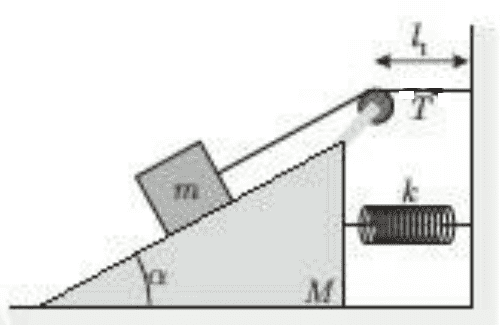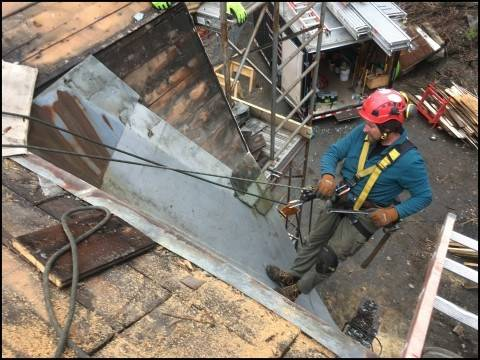# Motion of box on inclined plane connected by spring to a wall

Homework Statement:
See the picture below. Initially, the system is at rest. Neglecting friction and mass of both spring and string,
a) what is initial acceleration of M? Assume initially the spring is at natural length ##L_1##
b) find the tension when the system moves for the first time
c) is total energy of system conserved?
d) after moving a distance ##x_o## towards the wall, M will stop. Find ##x_o##
e) find the equilibrium point of M, measured from initial position of M, when M oscillates
f) find the period of oscillation of system
Relevant Equations:
∑F = m.a

Restoring force: F = k.x

ω = 2π / Ta) When the system is in motion for the first time, the force causing ##M## to move is contact force with ##m## so:
$$\Sigma F=M.a$$
$$N \sin \alpha=M.a$$
$$mg \cos \alpha \sin \alpha =M.a$$
$$a=\frac{mg \cos \alpha \sin \alpha}{M}$$

Is that correct?

b) Is acceleration of ##m## the same as ##M## when the system moves for the first time?

Thanks

haruspex
Homework Helper
Gold Member
2020 Award
Are you sure N=mg cos(α)? If so, in which direction will m accelerate in the ground frame? Which direction is that relative to M?

•songoku
Are you sure N=mg cos(α)?
Based on free body diagram (taking x-axis parallel to the slope of inclined plane), I think so

If so, in which direction will m accelerate in the ground frame? Which direction is that relative to M?
With respect to ground frame, ##m## will accelerate down the plane and ##M## to the right. With respect to ##M##, I think ##m## will also accelerate down the slope.

Or maybe my imagination is wrong? Actually with respect to ground, ##m## is accelerating vertically downwards and with respect to ##M##, ##m## is accelerating down the plane?

Thanks

haruspex
Homework Helper
Gold Member
2020 Award
Based on free body diagram (taking x-axis parallel to the slope of inclined plane), I think so
The FBD gives you the forces, but the equation is ΣF=ma. You are assuming m has no component of acceleration (in the ground frame) normal to the plane.
With respect to ground frame, m will accelerate down the plane and M to the right. With respect to M, I think m will also accelerate down the slope.
How can those three be true simultaneously?

•songoku
The FBD gives you the forces, but the equation is ΣF=ma. You are assuming m has no component of acceleration (in the ground frame) normal to the plane.

How can those three be true simultaneously?

I am not sure. Is there a certain way to determine the direction of acceleration of ##m## with respect to ground and relative to ##M##?

Thanks

Lnewqban
Gold Member
I am not sure. Is there a certain way to determine the direction of acceleration of ##m## with respect to ground and relative to ##M##?

Thanks
How much further down the sloped roof could this worker go?•songoku and Delta2
haruspex
Homework Helper
Gold Member
2020 Award
I am not sure. Is there a certain way to determine the direction of acceleration of ##m## with respect to ground and relative to ##M##?

Thanks
If the wedge has acceleration ##\vec a_w## and the mass has acceleration ##\vec a_{mw}## relative to the wedge, what is the mass's acceleration in the ground frame?

•songoku
How much further down the sloped roof could this worker go?View attachment 280370
All the way to the bottom of the slope? Sorry I don't really understand how to answer that question

If the wedge has acceleration ##\vec a_w## and the mass has acceleration ##\vec a_{mw}## relative to the wedge, what is the mass's acceleration in the ground frame?
##\vec a_m = \vec a_{mw} + \vec a_w##

Lnewqban
Gold Member
All the way to the bottom of the slope? Sorry I don't really understand how to answer that question
Yes, sorry.
I was just trying to tell you that there is no much freedom for the mass m to move away from the wall because the string, even if the pulley attached to mass M moves as far to the right as the spring allows M to move.

Why would M move against the resistance of the spring?

•songoku
haruspex
Homework Helper
Gold Member
2020 Award
##\vec a_m = \vec a_{mw} + \vec a_w##
Ok, so what equation can you write for the component of ##\vec a_{mw}## normal to the wedge?
What equation relates N and mg to the component of ##\vec a_m## normal to the wedge?

•songoku
Why would M move against the resistance of the spring?
Because there is contact force with ##m## that pushes ##M## to the right

Ok, so what equation can you write for the component of ##\vec a_{mw}## normal to the wedge?
What equation relates N and mg to the component of ##\vec a_m## normal to the wedge?
Should I first need to know the direction of ##\vec a_{mw}## and ##\vec a_m## to be able to answer this question?

Thanks

haruspex
Homework Helper
Gold Member
2020 Award
Because there is contact force with ##m## that pushes ##M## to the right

Should I first need to know the direction of ##\vec a_{mw}## and ##\vec a_m## to be able to answer this question?

Thanks
For my first question , does the mass lose contact with the wedge? Bury itself into the wedge? No? So what equation can you write for the component of ##\vec a_{mw}## normal to the wedge?

•songoku
For my first question , does the mass lose contact with the wedge? Bury itself into the wedge? No? So what equation can you write for the component of ##\vec a_{mw}## normal to the wedge?
I am not sure. I imagine the direction of ##\vec a_{mw}## is downwards parallel to the slope so the component of ##\vec a_{mw}## normal to the wedge is zero

haruspex
Homework Helper
Gold Member
2020 Award
I am not sure. I imagine the direction of ##\vec a_{mw}## is downwards parallel to the slope so the component of ##\vec a_{mw}## normal to the wedge is zero
Yes.

•songoku
What equation relates N and mg to the component of ##\vec a_m## normal to the wedge?
Is the direction of ##\vec a_m## vertically downwards?

Thanks

Lnewqban
Gold Member
If you observe the geometry of the string, you could see that:
1) The length of the RH portion of the string is reduced in the same magnitude that M moves towards the right.
2) The length of the LH portion of the string is increased in the same magnitude that M moves towards the right (because of 1 above); therefore, m slides down the slope and away from the pulley respect to M.

I believe that, respect to the ground, m moves downwards and towards the left as the spring gets compressed.

There is a reaction at the axis of the pulley due to the tension of the string, but perhaps its horizontal component is not significant enough to include it in the solution of this problem.

•songoku
haruspex
Homework Helper
Gold Member
2020 Award
Is the direction of ##\vec a_m## vertically downwards?

Thanks
Only if M=0.

Let me start again, laying out what I hope is a simpler path, working in scalars.
Let the acceleration of M be a.
Find a simple kinematic equation relating amw (which we know is parallel to the slope) to a.
Let the horizontal and vertical accelerations of m in the ground frame be ax, ay.
Write out the ΣF=ma equations in those directions. These will involve N, T and mg.
Write the horizontal ΣF=ma equation for M.

We need two more equations. These express ax, ay in terms of a, amw.
See how far you can get.

•songoku
I believe that, respect to the ground, m moves downwards and towards the left as the spring gets compressed.
You mean the direction of acceleration of m with respect to ground is parallel to the slope?

Let the acceleration of M be a.
Find a simple kinematic equation relating amw (which we know is parallel to the slope) to a.
You mean amw = am - a , where am is acceleration of m with respect to ground?

Thanks

haruspex
Homework Helper
Gold Member
2020 Award
You mean amw = am - a , where am is acceleration of m with respect to ground?

Thanks
No, just involving those two accelerations. Assume the whole string length is constant.

•songoku
haruspex
Homework Helper
Gold Member
2020 Award
respect to the ground, m moves downwards and towards the left
Whether it is left or right depends on α.

•songoku and Lnewqban
No, just involving those two accelerations. Assume the whole string length is constant.
Is it:

a = - amw cos α ?

Thanks

haruspex
Homework Helper
Gold Member
2020 Award
Is it:

a = - amw cos α ?

Thanks
I gave you the hint to think about string length. If the wedge moves a distance x towards the wall, what happens to the length of string from the pulley to the mass?

•songoku
I gave you the hint to think about string length. If the wedge moves a distance x towards the wall, what happens to the length of string from the pulley to the mass?
I tried to use that hint and also what Lnewqban posted in #16 but apparently I did not use it correctly.

When the wedge moves a distance x towards the wall, the pulley will also move as far as x towards the wall hence the length of string from the pulley to the wall decreases by x, so the length of string from the pulley to the mass increases by x since the total length should stay constant.

From there, I thought since ##a## is to the right and ##a_{mw}## is down the slope and the distance travelled by the mass and the wedge is the same, the magnitude of ##a## and ##a_{mw}## would be the same and I tried to find the component of ##a_{mw}## which is parallel to ##a##, hence ##a_{mw} \cos \alpha## directed to the left

Edit: Ah I see my mistake, ##|a|## is the same as ##|a_{mw}|## but is not the same as ##|a_{mw} \cos \alpha|## as ##|a_{mw} \cos \alpha|## will be less than ##a##

So the equation will be ##|a|=|a_{mw}|## ?

Thanks

•Lnewqban
haruspex
Homework Helper
Gold Member
2020 Award
So the equation will be |a|=|amw| ?
Yes.
So onto the next part...
Let the horizontal and vertical accelerations of m in the ground frame be ax, ay.
Write out the ΣF=ma equations in those directions. These will involve N, T and mg.
Write the horizontal ΣF=ma equation for M.

We need two more equations. These express ax, ay in terms of a, amw.

Last edited:
•songoku
Yes.
So onto the next part...
Let the horizontal and vertical accelerations of m in the ground frame be ax, ay.
Write out the ΣF=ma equations in those directions. These will involve N, T and mg.
Since ##a_m = a_{mw} + a## and for horizontal direction ##|a|> |a_{mw} \cos \alpha|##, I assume horizontally ##m## will move to the right with respect to the ground

∑F = m.ax
T cos α - N sin α = m.ax ....(1)

For vertical direction:
∑F = m.ay
mg - N cos α = m.ay ......(2)

Write the horizontal ΣF=ma equation for M.
∑F = M.a
N sin α = M.a .......... (3)

We need two more equations. These express ax, ay in terms of a, amw.
ax = a - amw .... (4)

ay = amw sin α .... (5)

Is that correct?

Thanks